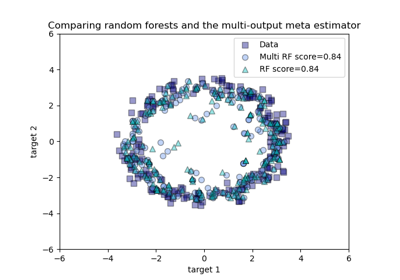# sklearn.multioutput.MultiOutputRegressor¶

class sklearn.multioutput.MultiOutputRegressor(estimator, *, n_jobs=None)[source]

Multi target regression

This strategy consists of fitting one regressor per target. This is a simple strategy for extending regressors that do not natively support multi-target regression.

New in version 0.18.

Parameters
estimatorestimator object

An estimator object implementing fit and predict.

n_jobsint or None, optional (default=None)

The number of jobs to run in parallel for fit. None means 1 unless in a joblib.parallel_backend context. -1 means using all processors. See Glossary for more details.

When individual estimators are fast to train or predict using n_jobs>1 can result in slower performance due to the overhead of spawning processes.

Changed in version v0.20: n_jobs default changed from 1 to None

Attributes
estimators_list of n_output estimators

Estimators used for predictions.

Examples

>>> import numpy as np
>>> from sklearn.multioutput import MultiOutputRegressor
>>> from sklearn.linear_model import Ridge
>>> clf = MultiOutputRegressor(Ridge(random_state=123)).fit(X, y)
>>> clf.predict(X[])
array([[176..., 35..., 57...]])


Methods

 fit(X, y[, sample_weight]) Fit the model to data. get_params([deep]) Get parameters for this estimator. partial_fit(X, y[, sample_weight]) Incrementally fit the model to data. Predict multi-output variable using a model score(X, y[, sample_weight]) Return the coefficient of determination R^2 of the prediction. set_params(**params) Set the parameters of this estimator.
__init__(estimator, *, n_jobs=None)[source]

Initialize self. See help(type(self)) for accurate signature.

fit(X, y, sample_weight=None, **fit_params)[source]

Fit the model to data. Fit a separate model for each output variable.

Parameters
X(sparse) array-like, shape (n_samples, n_features)

Data.

y(sparse) array-like, shape (n_samples, n_outputs)

Multi-output targets. An indicator matrix turns on multilabel estimation.

sample_weightarray-like of shape (n_samples,), default=None

Sample weights. If None, then samples are equally weighted. Only supported if the underlying regressor supports sample weights.

**fit_paramsdict of string -> object

Parameters passed to the estimator.fit method of each step.

Returns
selfobject
get_params(deep=True)[source]

Get parameters for this estimator.

Parameters
deepbool, default=True

If True, will return the parameters for this estimator and contained subobjects that are estimators.

Returns
paramsmapping of string to any

Parameter names mapped to their values.

partial_fit(X, y, sample_weight=None)[source]

Incrementally fit the model to data. Fit a separate model for each output variable.

Parameters
X(sparse) array-like, shape (n_samples, n_features)

Data.

y(sparse) array-like, shape (n_samples, n_outputs)

Multi-output targets.

sample_weightarray-like of shape (n_samples,), default=None

Sample weights. If None, then samples are equally weighted. Only supported if the underlying regressor supports sample weights.

Returns
selfobject
predict(X)[source]
Predict multi-output variable using a model

trained for each target variable.

Parameters
X(sparse) array-like, shape (n_samples, n_features)

Data.

Returns
y(sparse) array-like, shape (n_samples, n_outputs)

Multi-output targets predicted across multiple predictors. Note: Separate models are generated for each predictor.

score(X, y, sample_weight=None)[source]

Return the coefficient of determination R^2 of the prediction.

The coefficient R^2 is defined as (1 - u/v), where u is the residual sum of squares ((y_true - y_pred) ** 2).sum() and v is the total sum of squares ((y_true - y_true.mean()) ** 2).sum(). The best possible score is 1.0 and it can be negative (because the model can be arbitrarily worse). A constant model that always predicts the expected value of y, disregarding the input features, would get a R^2 score of 0.0.

Parameters
Xarray-like of shape (n_samples, n_features)

Test samples. For some estimators this may be a precomputed kernel matrix or a list of generic objects instead, shape = (n_samples, n_samples_fitted), where n_samples_fitted is the number of samples used in the fitting for the estimator.

yarray-like of shape (n_samples,) or (n_samples, n_outputs)

True values for X.

sample_weightarray-like of shape (n_samples,), default=None

Sample weights.

Returns
scorefloat

R^2 of self.predict(X) wrt. y.

Notes

The R2 score used when calling score on a regressor uses multioutput='uniform_average' from version 0.23 to keep consistent with default value of r2_score. This influences the score method of all the multioutput regressors (except for MultiOutputRegressor).

set_params(**params)[source]

Set the parameters of this estimator.

The method works on simple estimators as well as on nested objects (such as pipelines). The latter have parameters of the form <component>__<parameter> so that it’s possible to update each component of a nested object.

Parameters
**paramsdict

Estimator parameters.

Returns
selfobject

Estimator instance.

## Examples using sklearn.multioutput.MultiOutputRegressor¶